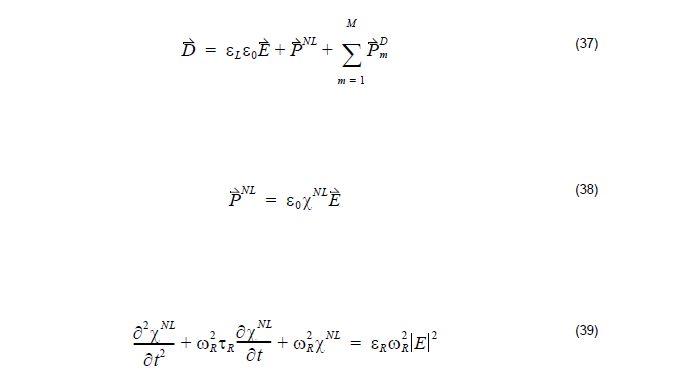Raman model allows another way to simulate the nonlinear phenomenon where the
nonlinear susceptibility was modeled by a second-order derivative equation which is
related to the resonant wavelength and the response timewhere

 εL is the linear relative permittivity XNL is the nonlinear susceptibility τ is the response time εR is Raman model permittivity ωR is the Raman model resonant frequency

In order to simulate the Raman effect, set four parameters for Raman model: εL, εR,
τ, and ωR.